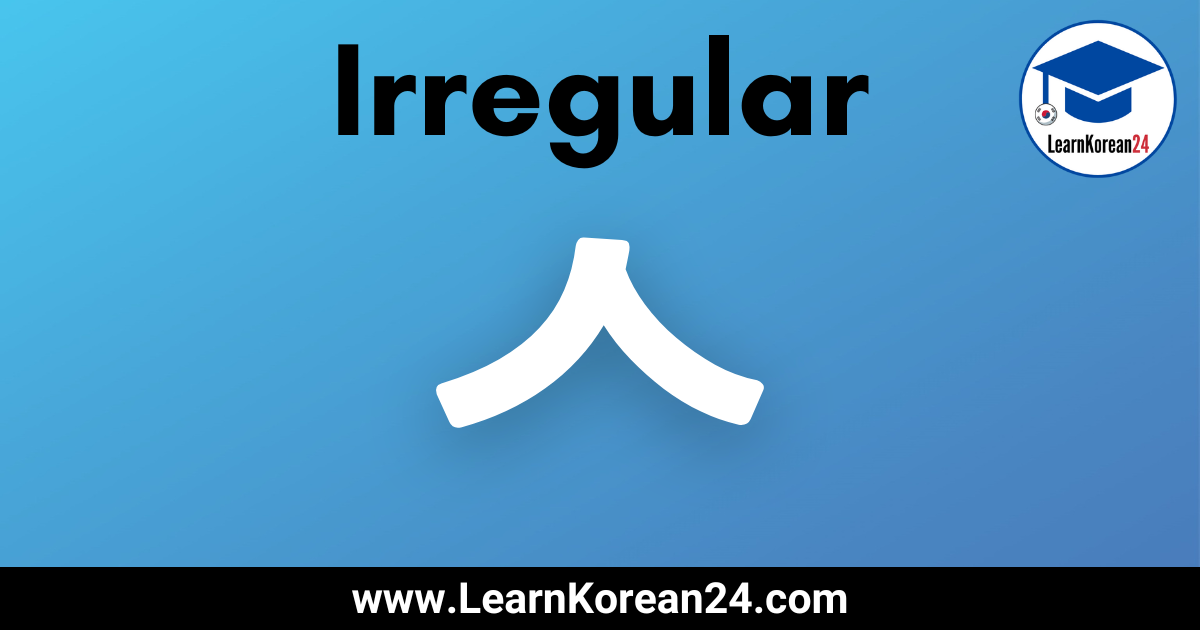# Irregular Korean Verbs With ㅅ (ㅅ 불규칙)In this lesson, you will learn about ㅅ irregular verbs (ㅅ 불규칙). In Korean, most verbs and adjectives which end in ㅅ are not irregular, and so they are conjugated the same as regular verbs and adjectives.

However, there are some verbs and adjectives which end in ㅅ that are irregular. As such, it is important to learn which Korean verbs ending in ㅅ are irregular, and how to conjugate ㅅ irregular verbs.

Below you will find a ㅅ irregular verb list and you will learn how to conjugate ㅅ irregular verbs.

## ㅅ Irregular Verbs (ㅅ 불규칙)

As was mentioned above, most verbs and adjectives which end in ㅅ are not irregular. In fact, there are only a few ㅅ irregular verbs. To help you learn which ㅅ verbs and adjectives are irregular we have put together a useful list of ㅅ irregular verbs.

### ㅅ Irregular Verbs List

• 긋다 = to draw
• 낫다 = to get well (verb) / to be better (adj)
• 붓다 = to pour / swell
• 젓다 = to stir
• 짓다 = to build
• 잇다 = to connect

## How To Conjugate ㅅ Irregular Verbs (ㅅ 불규칙)

Of the seven irregular verb forms in Korean, ㅅ irregular verbs are one of the easiest to learn as they have the fewest conjugation rules to remember.

Here are the conjugation rules to follow when conjugating ㅅ irregular verbs in Korean.

### Rule 1

Irregular ㅅ verbs and adjectives only behave irregularly when conjugated with endings that start with a vowel.

For example, endings such as 아요/어요 (present tense), ~(으)면 ( if), etc, start with a vowel, and so when these endings are attached to irregular ㅅ verbs, these verbs behave irregularly.

With other endings that start with a consonant, for example (e.g. (스)ㅂ니다, irregular ㅅ verbs and adjectives do not behave irregularly. That is, they are conjugated just the same as regular verbs.

### Rule 2

When conjugating ㅅ irregular verbs with endings that start with a vowel, ㅅ is always dropped from the verb stem.

For example, 긋다 (to draw) + 아요/어요 = 그어요. This is because ㅅ is dropped from the verb stem before adding 아요/어요.

### Rule 3

Vowels do not combine when conjugating ㅅ irregular verbs.

Usually when conjugating Korean words, when vowels meet, they are combined. For example, 마시다 (to drink) + 아요/어요 (present tense) = 마셔요 (NOT 마시어요). This is because the vowel from the verb stem and the vowel from the ending combine to make .

However, when conjugating irregular ㅅ verbs, the vowels do not combine. Here are some examples:

짓다 + 어요 = 지어요 (NOT 져요)
낫다 + 아요 = 나아요 (NOT 나요)

## ㅅ Irregular Examples

Here are some examples of ㅅ irregular verbs conjugated with some common endings. After reading these examples, if you’re not sure why they are conjugated in this way, please re-read the conjugation rules above.

As you can see in the table above, when the ㅅ irregular verbs are conjugated with endings which start with a vowel (아요/어요, (으)세요) the verb behaves irregularly. And so, ㅅ is dropped from the verb stem and the vowels do not combine.

With the ending starting with a consonant ( (스)ㅂ니다 ), the verbs do not behave irregularly. And so the verb stem does not change and ㅅ is not dropped.

## ㅅ Irregular Example Sentences

Here are some ㅅ Irregular example sentences:

• 한국에서는 아파트를 많이 지어요. (짓다) = In Korea, they build lots of apartments.
• 저는 책을 읽을 때 단어에 밑줄을 그어요. (긋다) = When I read books, I underline the words.
• 밤늦게 라면을 먹으면 다음날 얼굴이 부어요. (붓다) = If you eat noodles late at night, you will have a swollen face the next day.

## More Korean Irregular Verb Lessons

There are seven types of irregular verbs in Korean. For more lessons on Korean Irregular verbs, click on the links below.